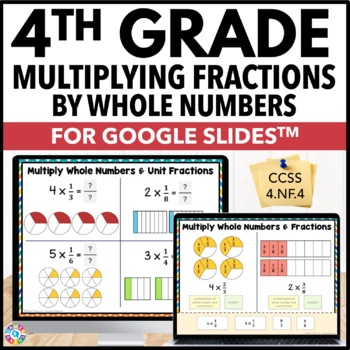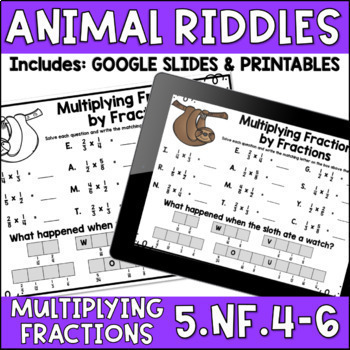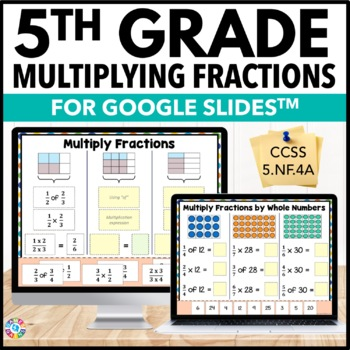Selected

#### Featured

Common Core Aligned
Social Emotional Learning
Bundles

Easel
PDF
Show all Formats

#### All Formats

Easel
Easel Activities
Easel Assessments
All Microsoft
Microsoft PowerPoint
Microsoft Word
Microsoft Excel
Microsoft Publisher
All Interactive Whiteboards
SMART Notebook
For SMART Board
ActiveInspire Flipchart
For Promethean Board
PDF
Image
Video
Audio
Internet Activities
e.g. Boom Cards
Prezi
Fonts

PreK
K
1st
2nd
3rd
4th
5th
6th
7th
8th
9th
10th
11th
12th

Other

Higher Education
Homeschool
Staff

#### Subjects

Arts & Music
English Language Arts
Foreign Language
Holidays/Seasonal
Math
Science
Social Studies - History
Specialty
For All Subject Areas

Free
Under \$5
\$5 - \$10
\$10 and up
On Sale

#### Resource Types

Independent Work Packet
Worksheets
Lesson
Printables
Activities
Assessment
Centers
Projects
Show all Resource Types

#### All Resource Types

Activboard Activities
Activities
Assessment
Bibliographies
Bulletin Board Ideas
By TpT Sellers for TpT Sellers
Centers
Classroom Forms
Clip Art
Cultural Activities
English (UK)
Flash Cards
For Parents
Games
GATE
Grant Proposals
Graphic Organizers
Handouts
Homeschool Curricula
Homework
Independent Work Packet
Interactive Notebooks
Laboratory
Lectures
Lesson
Literature Circles
Microsoft OneDrive
Montessori
Movie Guides
Outlines
Posters
Printables
Professional Development
Professional Documents
Projects
Reflective Journals for Teachers
Research
Rubrics
Scaffolded Notes
Scripts
Simulations
Songs
Study Guides
Syllabi
Teacher Manuals
Thematic Unit Plans
Unit Plans
Webquests
Word Walls
Workbooks
Worksheets

# Results for multiply fractions

37,000+ results## 4.NF.3 & 4.NF.4 Practice: Add, Subtract, & Multiply Fractions

This CCSS aligned fraction packet includes 22 practice sheets filled with practice fraction problems, example problems, and step-by-step directions. These can be used to introduce a concept, review a concept, or send home as reinforcement for homework. An answer key is provided. - - - - - - - - - - - - - - - - - - - - - - - - - - - - - - - - - - - - - - - - - - - - - - - - - - - - - - - - - - - - - - - - - - -This resource can be used along with our other 4.NF.3 &amp; 4.NF.4 resources:4.NF.3 &am## 5th Grade Fraction Center Games - Add, Subtract, Multiply & Divide Fractions...

Motivate your students with these NO-PREP games! Included are 14 engaging games, which are perfect for practicing key 5th grade fraction standards: adding fractions, subtracting fractions, multiplying fractions, dividing fractions, and more! These games support Common Core standards 5.NF.1, 5.NF.3, 5.NF.4, 5.NF.5, and 5.NF.7.This pack includes 14 print-and-play games that cover the following 5th grade fraction skills:Adding and Subtracting FractionsAdding proper fractions with unlike denominator## Fraction Riddles: Add, Subtract, Multiply, and Divide

Add, subtract, multiply, and divide fractions to solve riddles. Included:6 worksheets with answer keysAdding and SubtractingMultiplyingDividingMultiplying and Dividing (two fractions)Multiplying and Dividing (fraction and whole number)All four operations*****************************************************************************Check out all of my fraction resourcesCheck out all of my math riddles****************************************************************************Common Core State Stan## Multiplying Fractions Task Cards | Digital and Printable

A set of 40 digital and printable multiplying fractions task cards using visual models, perfect for differentiation! These printable and digital cards use a variety of different ways to challenge and engage your students in multiplication tasks. Task cards are a great tool for differentiation - simply choose best card(s) for each student! These multiplying fractions task cards include visual models, word problems, simple equations, and more! Check out the preview for a look at the different typ## Multiply and Divide Fractions Activity | Battle My Math Ship Game

This no-prep, partner activity is a fun spin on Battleship! This math review game will have your students begging for more activities just like it! Students take turns choosing spaces to attack by multiplying and dividing fractions. There are no mixed numbers that are multiplied or divided. This activity includes PRINT and DIGITAL versions!Please see the preview to view problems used in the activity.Check out more battle my math ship activities here or get all of them...the ULTIMATE BATTLESHIP B## Multiplying and Dividing Fractions | Coloring Activity

Multiplying and Dividing Fractions Coloring ActivityThis is a fun way for students to practice multiplying and dividing fractions. There are 20 problems total, separated into two sets. Students find each product or quotient, then look for matching answers between the sets. They color the "I ♥ Math" picture according to their matching answers. This could also used as a partner activity in which Partner A does Set 1 and Partner B does Set 2.All positive fractions. Answers given as mixed fracti## Rational Number Operations Unit | Add, Subtract, Multiply, & Divide Fractions

A 12 day CCSS-Aligned Rational Number Operations Unit including: adding, subtracting, multiplying, and dividing rational numbers (both positive and negative). Students will use the additive inverse property, number line models, and the algorithm to solve rational number problems.Students will practice with both skill-based problems, real-world application questions, and error analysis to support higher level thinking skills. You can reach your students and teach the standards without all of the## Multiplying and Dividing Fractions

This Multiplying and Dividing Fractions Resource contains a variety of printables to help you teach the math skills of multiplying whole numbers by fractions, fractions by fractions, dividing whole numbers by unit fractions, and dividing unit fractions by whole numbers in a conceptual way.Click here to see a BUNDLED resource that includes this resource and SIX other 5th Grade Common Core math resources at a discounted price!The following resources are included in this Multiplying and Dividing Fr## Multiply and Divide Fractions Task Cards & Game with Word Problems Math Review

Multiplying and dividing fractions can be fun with these Multiply and Divide Fractions Task Cards. Includes straight computation and word problems! Play as a game with the included game-board during stations, use for scoot in whole, or use with almost any game in small group! They are so engaging and versatile and perfect for practice of this skill!Covers topics such as:*word problems*multiply fraction by fraction*multiply mixed number by fraction*multiply whole number by fraction*dividing fr## 5.NF.4 & 5.NF.6 Practice Sheets: Multiply Fractions

This 5.NF.4/6 CCSS aligned fraction packet includes 26 practice sheets to teach/reinforce multiplying fractions including fractions x whole numbers, fractions x fractions, and fractions x mixed numbers. These sheets can be used to introduce a concept, review a concept, or send home as reinforcement for homework. An answer key is provided.------------------------------------------------------------------------------------------------------------Check out our other 5.NF.4&amp;6 resources:5.NF.4 TaGoogle Paperless Practice - 4th Grade Multiplying Fractions by Whole Numbers {4.NF.4, 4.NF.4A, 4.NF.4B, 4.NF.4C}Engage your students with this interactive DIGITAL resource that works with Google Slides™. No more copies to be made, no more printer ink, and no more lost papers! With this 24-slide digital resource, your students will practice multiplying fractions by whole numbers and representing fractions as products of a whole number times a unit fraction. Students will love interacting with t## 4.NF.3 & 4.NF.4 Bundle: Add, Subtract, & Multiply Fractions/Mixed Numbers

This bundle has everything you need to teach 4.NF.3 and 4.NF.4: Add, Subtract, &amp; Multiply Fractions and Mixed Numbers. This includes 22 practice sheets, 2 assessments, 19 learning posters, 36 task cards, and 2 matching activities! Buy the bundle and save 15%! :) WOW!Click on each link below to see each bundle item's preview.- - - - - - - - - - - - - - - - - - - - - - - - - - - - - - - - - - - - - - - - - - - - - - - - - - - - - - - - - - - - - - - - - - -This bundle includes these 6 resource## MULTIPLY & DIVIDE FRACTIONS Maze, Riddle, Coloring | Google | Distance Learning

Have your students apply their understanding of MULTIPLYING &amp; DIVIDING FRACTIONS with these fun activities including a maze, riddle, and coloring activity. What Is Included:1. Multiply &amp; Divide Fractions COLORING ACTIVITY (pdf)Students are prompted to MULTIPLY &amp; DIVIDE FRACTIONS. Students use their solutions to color the picture. 2. Multiply and Divide Fractions MAZE (pdf and digital)This is a self-checking worksheet that allows students to strengthen their understanding of MULTIPLYI## Multiply Fractions, Whole Numbers, Mixed Numbers Riddles Worksheets Google Slide

This packet includes four zoo animal themed riddle worksheets. Each worksheet is designed for you to use as a review for multiplying fractions, whole numbers, and/or mixed numbers (5.NF, 4, 5.NF.5, &amp; 5.NF.6)! It can be printed or used digitally in Google Classroom or in programs that Microsoft PowerPoint, such as Microsoft Teams! You will download this product straight to your Google Drive. The download will include the Google Slides you can assign to your students on Google Classroom, as we## 5th Grade Multiplying Fractions by Whole Numbers & Fractions {5.NF.4} Google

Google Paperless Practice - 5th Grade Multiplying Fractions by Whole Numbers &amp; Fractions {5.NF.4, 5.NF.4A}Engage your students with this interactive DIGITAL resource that works with Google Slides™. No more copies to be made, no more printer ink, and no more lost papers! With this 24-slide digital resource, your students will practice multiplying fractions by whole numbers and multiplying fractions by fractions. Students will love interacting with the movable pieces and typing their response## Multiply Fractions by Whole Numbers Whodunnit Activity -Printable & Digital Game

Nothing like a good criminal investigation to liven up multiplying fractions by whole numbers! Based off my popular CSI projects, I have created Whodunnits? The Whodunnits plan to focus on single content topics as opposed to units and will focus more on skill building than application. Every day can't be a project day, so Whodunnits can be an engaging out of their seat activity to practice important skills. In a Whodunnit?, students will work in teams to solve 10 practice problems that increase## Multiplying and Dividing with Fractions & Mixed Numbers Word Problems {No Prep}

Multiplying and Dividing with Fractions and Mixed Numbers Word Problem RTI No Prep Resource! This resource aligns the 5th grade Common Core Standards 5.NF.6 and 5.NF.7.Click here for the bundle of all 5 of my Word Problem No Prep Intervention Resources for 5th Grade.Word Problem PracticeThis resource includes 30 word problems that focus on the following skills:Multiplying Fractions by Whole NumbersMultiplying FractionsMultiplying with Mixed NumbersDividing with Whole Numbers and Unit FractionsOn## Multiplying Fractions Color by Number Print and Digital

This multiplying fractions activity has you covered with both print and digital versions! Students answer 20 fraction multiplication problems and then find the solution numbers on the coloring page/section and color with the colors indicated.Check out the Preview above to see what's included and to see a demo of the digital version.Problems require students to:Multiply fractions by fractions (13 problems)Multiply fractions by whole numbers (7 problems) In this easy-prep resource for multiplying## Multiplying Fractions Using Area Models Practice Packet (Common Core Aligned)

Multiplying fractions is a key part of the fifth grade mathematics curriculum. This ten-page packet focuses on helping students develop a conceptual understanding of what it means to multiply two fractions. The packet provides scaffolded instruction and practice, first by reviewing what it means to "multiply" two numbers, and then applying that definition to multiplying two fractions. The packet uses area models to provide a visual for students of what occurs when two fractions are multiplied## Emoji Multiplying and Dividing Fractions | Fractions Color by Number

These emoji multiplying and dividing fractions mystery pictures will provide lots of fun practice for your students! Students solve math problems and color by code. This set of math mysteries reviews the following:Multiplying a fraction by a whole numberMultiplying a fraction by a fractionDividing unit fractions by whole numbersDividing whole numbers by unit fractionsDividing fractions by fractionsThese math mysteries are great for:Math CentersEarly FinishersHomeworkThe following Common Core S## Multiplying and Dividing Fractions Game Show | 5th Grade Math Test Prep Activity

Review Multiplying and Dividing Fractions in a fun and interactive way that involves your whole class. This engaging game showplays like Jeopardy® and covers 5th grade math skills, including multiply whole numbers and fractions, multiply fractions by fractions, multiply mixed numbers, divide whole numbers by unit fractions, and divide unit fractions by whole numbers. Perfect for an end of year or end of unit review.⭐ GET THE 5TH GRADE REVIEW BOWL BUNDLE &amp; SAVE ⭐Save 30% by purchasing this re## Multiplying Fractions by a Whole Number Word Problem Task Cards - Set of 28

This set of 28 task cards allows students to practice multiplying fractions by a whole number in word problems. These task cards are aligned to 4th and 5th grade Common Core standards. Included: - set of 28 task cards - student recording sheet - answer key These task cards cover Common Core standards: CCSS.Math.Content.4.NF.B.4c Solve word problems involving multiplication of a fraction by a whole number, e.g., by using visual fraction models and equations to represent the problem. CCSS.Ma## Multiplying Fractions Math Pennant Activity

Students multiply fractions using the area model, then hang their finished work in your classroom as colorful student-created classroom décor! Once a pennant is complete, it can be hung along a string in your classroom to show the world that, "Hey, we know how to multiply fractions!"Included are 20 pennants with fraction multiplication problems, an optional student answer sheet and an answer key. Each group of students can be given a set of pennants, crayons, scissors and either glue, tape or a## Multiplying Fractions Doodle Notes

Fraction Multiplication: "doodle notes" - When students color or doodle in math class, it activates both hemispheres of the brain at the same time. There are proven benefits of this cross-lateral brain activity: - new learning - relaxation (less math anxiety) - visual connections - better memory &amp; retention of the content! Students fill in the sheets, answer the questions, and color, doodle or embellish. Then, they can use it as a study guide later on. Content includes: - Fraction time
Showing 1-24 of 37,000+ results